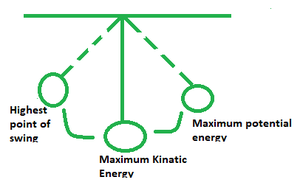# Conservation of Energy Formula

• Last Updated : 14 Mar, 2022

Energy can be defined as the ability to perform work in physics. Law of Conservation of Energy states that energy cannot be made or destructed in any process. It may, however, be changed from one form to another. The overall energy contained in the isolated system remains constant when all kinds of energy are considered. This law applies to all kinds of energy. To sum it up:

A single system, shut down from all its surroundings, retains its complete energy. This is illustrated through the following diagram of a swinging pendulum:Formula

Ut = Ui + W + Q

where,

• Ui denotes the initial energy of a system
• W denotes the work done
• Q denotes the heat added or removed

Derivation

Assuming that the gravitational potential at the earth’s surface is zero. Consider the case of a fruit falling to the ground.

Assume A to be a point on the tree at a height of ‘h’ from the ground; the fruit’s velocity is zero, hence energy is highest there.

E = mass × gravitation constant × height   …(1)

Along the path of its descent, its potential energy diminishes but its kinetic energy grows.

The fruit is falling freely under gravity towards the bottom of the tree at point B, and it is at a height ‘a’ from the ground, and it has speed as it reaches point B. As a result, it will have simultaneously kinetic and potential energy at this moment.

Potential energy = m × g × x        …(2)

Third equation of motion states that: v2 = 2g × (h – a)

or, Kinetic energy of the fruit = mg × (h – a)            …(3)

From the above equations, we have:

E = mg × (h – a) + m × g × x

= mgh

Similarly the result can be proved for different value of height of the falling fruit.

### Sample Problems

Question 1. An object of 2 kg is dropped from a 30 m building. Find its velocity when it is 10 m above the ground.

Solution:

The object’s potential energy decreases as it falls, but its kinetic energy increases.

Change in potential energy = Final mgf – Initial mgf

= 2 kg x 10 m/s x 10 m – 2 kg x 10 m/s x 30 m

= −400 J

Now, mv2/2 = 400

or, v = 20 m/s

Question 2. Why are perpetual motion devices unable to function?

Solution:

The perpetual motion machine is an idea for a machine that continues to move indefinitely without slowing down. Over the years, an endless variety of wondrous equipment have really been described. Pumps that are said to run themselves using their own top of water falling, wheels that are said to move themselves using unbalanced masses, and a variety of self-repelling magnets are among them.

Question 3. Find the energy conserved in a system given its initial energy 600 J and final energy 4800 J.

Solution:

ΔEsys​ =  Ein​−Eout

= 4800 – 600 J

= 4200 J

Question 3. When would a spring undergoing motion have the highest kinetic energy? Explain.

Solution:

When the spring is travelling at its quickest, the kinetic energy is at its maximum. When the spring is most squeezed and briefly immobile, however, potential energy is maximum. When the spring extends due to the force of compression, potential energy falls as velocity increases.

Question 4. A pendulum of mass 390 kg has reached its maximum height at 1.2 m. Find its velocity at the lowest point.

Solution:

Potential energy of the pendulum at a height of 1.2 m = mgh

= 390 x 10 x 1.2

= 4680 J

Now, 4680 J = mv2/2

v = 4.88 m/s

Question 5. An object of 12 kg is dropped from a 60 m building. Find its velocity when it is 10 m above the ground.

Solution:

The object’s potential energy decreases as it falls, but its kinetic energy increases.

Change in potential energy = Final mgf – Initial mgf

= 12 kg x 10 m/s x 10 m – 12 kg x 10 m/s x 60 m

= 1200 – 7200

= −6000 J

Now, mv2/2 = 6000

or, v = 10 m/s

My Personal Notes arrow_drop_up